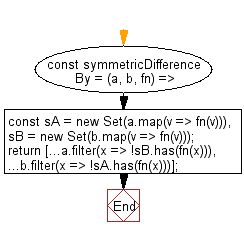# JavaScript: Get the symmetric difference between two given arrays, after applying the provided function to each array element of both

## JavaScript: Exercise-133 with Solution

Write a JavaScript program to get the symmetric difference between two given arrays, after applying the provided function to each array element of both.

• Create a new Set() from each array to get the unique values of each one after applying fn to them.
• Use Array.prototype.filter() on each of them to only keep values not contained in the other.

Sample Solution:

JavaScript Code:

``````//#Source https://bit.ly/2neWfJ2
const symmetricDifferenceBy = (a, b, fn) => {
const sA = new Set(a.map(v => fn(v))),
sB = new Set(b.map(v => fn(v)));
return [...a.filter(x => !sB.has(fn(x))), ...b.filter(x => !sA.has(fn(x)))];
};
console.log(symmetricDifferenceBy([2.1, 1.2], [2.3, 3.4], Math.floor));
```
```

Sample Output:

```[1.2,3.4]
```

Flowchart:Live Demo:

See the Pen javascript-basic-exercise-133-1 by w3resource (@w3resource) on CodePen.

Improve this sample solution and post your code through Disqus

What is the difficulty level of this exercise?

Test your Programming skills with w3resource's quiz.

﻿